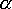QUESTION #33

# What is the fine-structure constant?

The fine-structure constant is a unitless numerical constant - whose value is approximately equal to: 1/137. In fact the exact value of the fine-structure constant is: 0.007297351 +/- 0.000000006. Accepted symbol for the fine-structure constant is Greek alpha (). [Ref. 1]

What is the physical origin of this constant? Well, it is related to the so called Fine Structure - closely spaced groups of optical spectrum lines of elements like: hydrogen and helium. These optical spectrum lines are in a way the fingerprint of the element's energy levels. Since the energy levels of any given element are quantized (i.e. only discrete energy levels are available) the optical spectrum appears as a series of lines instead of a continuous spectrum. With each such optical spectrum line one can associate three numbers:

n - the principal quantum number
l - the azimuthal quantum number
j - the angular momentum quantum number
(an additional quantum number also can be considered that will give rise to spin-orbit interaction in atoms with more than one electron)

Each group of spectral optical lines mentioned above has a same n - number, but different values of l and j numbers. According to P.A.M. Dirac's relativistic quantum mechanics, energy levels of a one-electron atom (hydrogen is a good example) which have the same n and j numbers will coincide exactly - but their value will be different from that predicted by the Bohr's theory by an amount that is proportional to the square of the fine-structure constant (alpha).

There have been deviations from Dirac's theory discovered in 1947. Namely, the level having l=0 does not coincide with that having l=1. This discrepancy was later named the Lamb shift (after its discoverer Willis Lamb, Jr.) and is due to the interaction of an electron with the zero-point fluctuations of the electromagnetic field.

As mentioned above in a multi electron atom systems the spin-orbit interaction becomes important too and the number of optical spectrum lines in the groups becomes even more numerous.

Update: August 2001 - Evidence published in the Physical Review Letters that the fine structure constant may be slightly different to what we believed. Click here for the full article.

Ref. 1: McGraw-Hill Encyclopedia of Physics, Second Edition